# Temperature Calculation

At every Time Step during the simulation, Universe Sandbox calculates the change in the surface temperature across the surface of every Planetary Body object in the simulation. These calculations consider the effects of incoming heat from sources like nearby stars, the effects of cooling, and the spread of heat across the surface of the object.

## Related Properties & Settings

### Properties

• The temperature of each point on the object's surface can be seen by hovering over that spot on the Temperature surface map.
• The Average Surface Temperature property of an object represents the average temperature over the entire surface of the object.
• The Minimum Temperature and Maximum Temperature represent the lowest and highest temperatures on the Temperature map, respectively.
• Several properties related to the movement of energy into and out of the object, and the effect of this energy flow on temperature, are listed in the Energy Flow section of the object's properties panel.

## Models

### Energy Flow

The temperature of an object depends on the flow of energy in and out of the object. The rate at which energy is absorbed and radiated by the object, also known as the power, will affect the rate at which the temperature changes.

For each point on the surface map of the object, Universe Sandbox performs the following calculations in each time step. First, Universe Sandbox calculates the rate of energy absorbed from various sources in the simulation (listed below). The total Energy Absorption Rate, or absorption power, is the sum of the the rates from each source: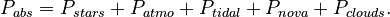$P_{abs} = P_{stars} + P_{atmo} + P_{tidal} + P_{nova} + P_{clouds}.$

The power absorbed by an object can come from a number of sources, including:

In each time step, the each point on the object's surface will also radiate energy out into space. The Energy Radiation Rate, or radiation power, is calculated from the temperature of the point using the Stefan-Boltzmann law: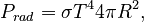$P_{rad} = \sigma T^4 4 \pi R^2,$

where σ is the Stefan-Boltzmann constant and R is the radius of the object.

### Surface Temperature

The change in the temperature of each point on the object's surface, ΔT, is calculated using the Energy Absorption Rate, Pabs, the Energy Radiation Rate, Prad, the time step, Δt, and the object's Surface Heat Capacity, C, using$\Delta T = (P_{abs} - P_{rad})\Delta T / C.$

Heat flows from areas of high temperature to areas of low temperature, so the heat in a single point on the surface map of an object may spread or diffuse into nearby points, and the heat in those points may spread to the first point. Universe Sandbox calculates this diffusion by using the FTCS method to solve the heat equation. The speed of diffusion, which in reality depends on the material that makes up the surface, is determined by the simulation's Heat Diffusion Rate. The temperatures of each point on the surface map are then corrected based on the results of this diffusion calculation.

The Average Surface Temperature is calculated by averaging the surface temperatures of each point on the surface, weighted by the area of each point.

### Equilibrium and Effective Temperatures

As described above, the change of temperature in each time step depends on the flow of energy into and out of the object. When the absorbed power exactly equals the radiated power, the object is in thermal equilibrium, and its temperature will not change. Since the radiated power depends on the temperature of the object, there is a certain temperature where this equilibrium occurs,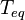$T_{eq}$. At the Equilibrium Temperature, the radiated power equals the absorbed power: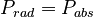$P_{rad} = P_{abs}$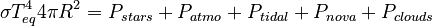$\sigma T_{eq}^4 4 \pi R^2 = P_{stars} + P_{atmo} + P_{tidal} + P_{nova} + P_{clouds}$

The Effective Temperature is the equilibrium temperature that the object would have if it were a perfect blackbody. In the case of the Universe Sandbox simulation, this means the equilibrium temperature the object would have without an atmosphere: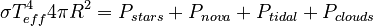$\sigma T_{eff}^4 4 \pi R^2 = P_{stars} + P_{nova} + P_{tidal} + P_{clouds}$

If the object does not have an atmosphere, the Equilibrium Temperature will equal the Effective Temperature.

## Limitations

### Heating/Cooling

The rate at which an object radiates energy into space depends strongly on its temperature (in fact, it depends on temperature to the fourth power). But the rate at which the temperature changes depends on the energy radiation rate. This makes it challenging to accurately predict what the temperature of an object will be far in the future. Instead, Universe Sandbox approximates the temperature change as a linear change in each time step. This is similar to how Universe Sandbox approximates the motion of objects as being linear in each time step in the N-Body Simulation. As with the N-body simulation, this approximation works best for small time steps and slow rates of change.

At large time steps and/or large heating and cooling rates, the temperature calculations described above will become less accurate. Most notably, the errors in the temperature calculations for each point may affect the Average Surface Temperature, leading to errors in the Heating Rate.

### Heat Diffusion

The simulation of heat spreading from hotter regions of the surface to cooler regions also depends on the time step. At higher time steps, the FTCS method that Universe Sandbox uses to solve the heat equation becomes unstable and extremely inaccurate. At these higher time steps, Universe Sandbox will artificially lower the speed of heat diffusion to avoid instability in the solution.